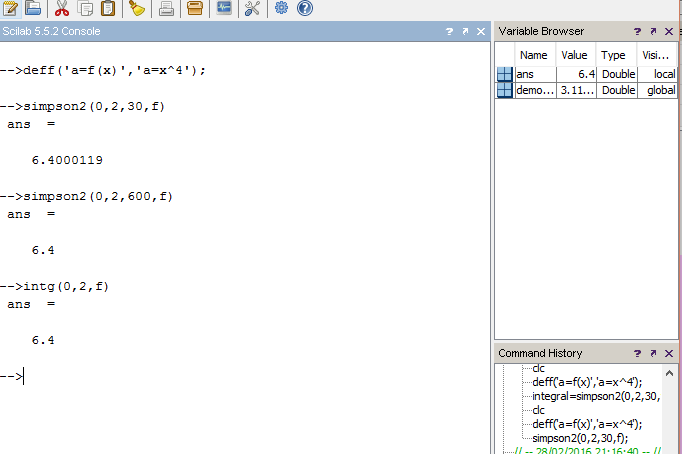# Simpson’s 3/8th Rule Integration SCILAB CODE(Program/Macro)

The following is the code for evaluating a definite integral of a given function by a Numerical Method called Simpson’s 3/8th Rule.

```//Simpson's 3/8th Rule
//Evaluates the definite integral of a function f(x), from a to b.
//Written By: Manas Sharma(www.bragitoff.com)
funcprot(0);
function ans=simpson2(a,b,n,f)//function definition of simpson
h=(b-a)/n;
sum=0;
for i=1:n-1
x=a+i*h;
if modulo(i,3)==0
sum=sum+2*f(x);
else
sum=sum+3*f(x);
end
end
ans=(3*h/8)*(f(a)+f(b)+sum);
endfunction
//NOTE: When the function is called the value of the third argument that is, n, should be a multiple of 3.

```

You can either copy the code above and save it as a .sci file or download the file . Once you run the code, the function ‘simpson2(a,b,n,f)’ can be called by other programs or even in the console.

Function syntax:

simpson2(a,b,n,f)

where,

a=initial limit(real no.)
b=final limit(real no.)
n=no. of sub-intervals(the higher the value of ‘n’ the better is the result.
NOTE: n should be a multiple of 3.

Example:
The following code snippet evaluates the integral of 1/(1+x^2) from 0 to 2.[wpedon id="7041" align="center"]